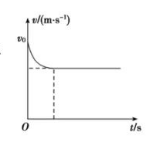$\text{A.}$ 汽车再次匀速运动时速度大小为 $\frac{2 v 0}{3}$ $\text{B.}$ $t=0$ 时刻, 汽车的加速度大小为 $\frac{P}{3 m v 0}$ $\text{C.}$ 汽车的牵引力不断减小 $\text{D.}$ 经历的时间为 $\frac{3 s}{2 v 0}$